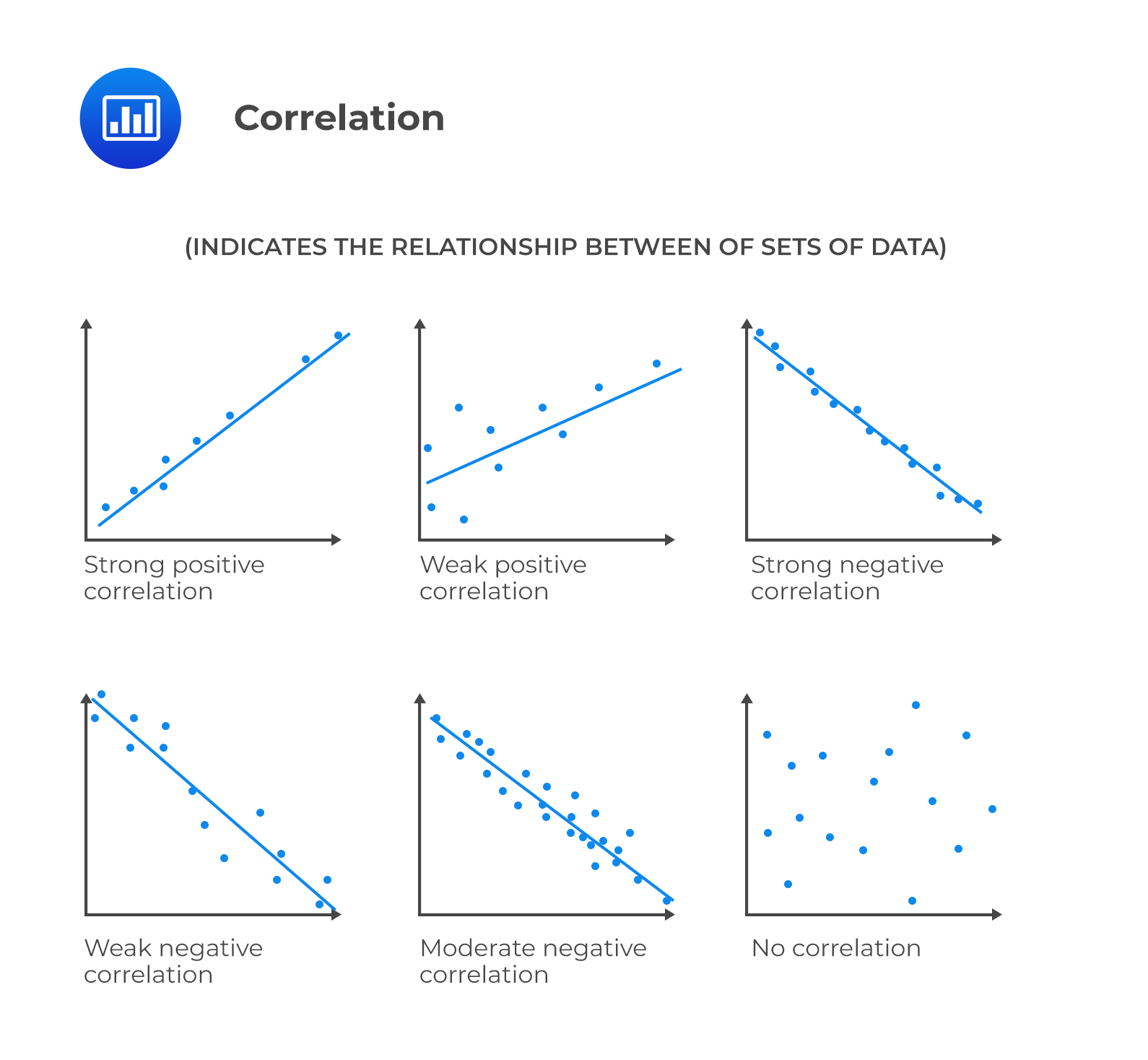# Correlation

## Covariance

Covariance is a measure of how two variables move together. The sample covariance of X and Y is calculated as follows:

$$\mathrm{S}_{\mathrm{XY}}=\frac{\sum_{\mathrm{i}=1}^{\mathrm{N}}\left(\mathrm{X}_{\mathrm{i}}-\overline{\mathrm{X}}\right)\left(\mathrm{Y}_{\mathrm{i}}-\overline{\mathrm{Y}}\right)}{\mathrm{n}-1}$$

A major drawback of covariance is that it is difficult to interpret since its value can vary from negative infinity to positive infinity.

## Correlation

Correlation is a measure of the linear relationship between two variables. It takes the covariance and divides it by the product of the standard deviations of both variables. As a result, its value ranges between -1 and +1 and is easier to interpret.

The sample correlation coefficient is calculated as follows:

$$r_{X Y}=\frac{s_{X Y}}{s_{x} \times s_{y}}$$

Where:

$$s_{X Y}$$ = Covariance between variable X and Y.

$$s_{X}$$ = Standard deviation of variable X.

$$s_{Y}$$ = Standard deviation of variable Y.### Properties of Correlation

• Correlation ranges between −1 to +1 for two random variables, X and Y.
• A correlation of 0 (uncorrelated variables) indicates no linear (straight line) relationship exists between the variables.
• A positive correlation close to +1 indicates a strong positive linear relationship.
• A correlation of 1 indicates a perfect linear relationship.
• A negative correlation close to −1 indicates a strong negative linear relationship.
• A correlation of −1 indicates a perfect inverse linear relationship.

### Limitations of Correlation Analysis

• Two variables can have a very low correlation despite having a strong nonlinear relationship.
• Correlation can be an unreliable measure when outliers are present in the data.
• Correlation does not imply causation. This implies that correlation may be spurious. A spurious correlation refers to:
• correlation between two variables due to chance relationships in a particular dataset;
• correlation arising between variables when they are divided by a third variable; or
• correlation between two variables arising from their relation to a third variable.

## Question

The correlation coefficient between X and Y is 0.7 and the covariance is 29. If the variance of Y is 25, the variance of X is closest to:

1. 8.29.
2. 29.
3. 68.65.

Solution

\begin{align} r_{X Y} &=\frac{s_{X Y}}{s_{X} \times S_{Y}}\\ \Rightarrow 0.7 &=\frac{29}{X \bullet 5} \\ \therefore X&=8.2857\\ \\ \text{Variance} &=8.2857^2=68.65 \end{align}

Shop CFA® Exam Prep

Offered by AnalystPrepLevel I
Level II
Level III
All Three Levels
Featured Shop FRM® Exam PrepFRM Part I
FRM Part II
FRM Part I & Part II
Learn with Us

Subscribe to our newsletter and keep up with the latest and greatest tips for success
Shop Actuarial Exams PrepExam P (Probability)
Exam FM (Financial Mathematics)
Exams P & FM
Shop GMAT® Exam PrepComplete CourseSergio Torrico
2021-07-23
Excelente para el FRM 2 Escribo esta revisión en español para los hispanohablantes, soy de Bolivia, y utilicé AnalystPrep para dudas y consultas sobre mi preparación para el FRM nivel 2 (lo tomé una sola vez y aprobé muy bien), siempre tuve un soporte claro, directo y rápido, el material sale rápido cuando hay cambios en el temario de GARP, y los ejercicios y exámenes son muy útiles para practicar.diana
2021-07-17
So helpful. I have been using the videos to prepare for the CFA Level II exam. The videos signpost the reading contents, explain the concepts and provide additional context for specific concepts. The fun light-hearted analogies are also a welcome break to some very dry content. I usually watch the videos before going into more in-depth reading and they are a good way to avoid being overwhelmed by the sheer volume of content when you look at the readings.Kriti Dhawan
2021-07-16
A great curriculum provider. James sir explains the concept so well that rather than memorising it, you tend to intuitively understand and absorb them. Thank you ! Grateful I saw this at the right time for my CFA prep.nikhil kumar
2021-06-28
Very well explained and gives a great insight about topics in a very short time. Glad to have found Professor Forjan's lectures.Marwan
2021-06-22
Great support throughout the course by the team, did not feel neglectedBenjamin anonymous
2021-05-10
I loved using AnalystPrep for FRM. QBank is huge, videos are great. Would recommend to a friendDaniel Glyn
2021-03-24
I have finished my FRM1 thanks to AnalystPrep. And now using AnalystPrep for my FRM2 preparation. Professor Forjan is brilliant. He gives such good explanations and analogies. And more than anything makes learning fun. A big thank you to Analystprep and Professor Forjan. 5 stars all the way!michael walshe
2021-03-18
Professor James' videos are excellent for understanding the underlying theories behind financial engineering / financial analysis. The AnalystPrep videos were better than any of the others that I searched through on YouTube for providing a clear explanation of some concepts, such as Portfolio theory, CAPM, and Arbitrage Pricing theory. Watching these cleared up many of the unclarities I had in my head. Highly recommended.